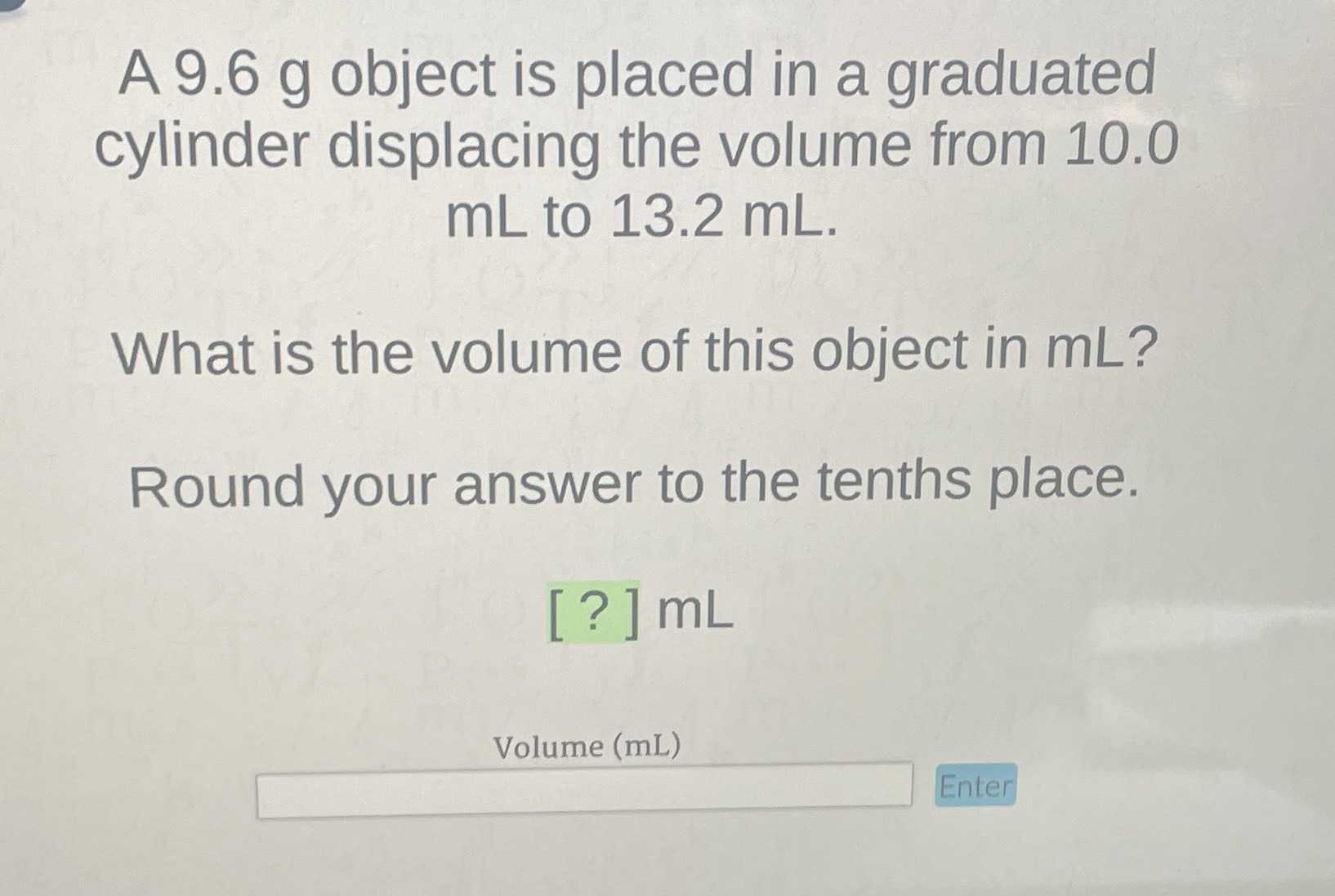### Still have math questions?

Arithmetic
Question$$9.6 g$$ object is placed in a graduated cylinder displacing the volume from $$10.0$$ $$mL$$ to $$13.2 mL .$$

What is the volume of this object in $$mL ?$$ Round your answer to the tenths place. [?] $$mL$$